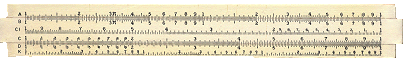## Analog Scientific Calculator

For more than 350 years the standard tool for scientific calculations was the slide rule.

Not long after John Napier discovered logarithms in 1614, William Oughtred invented several slide rules to simplify multiplication. In 1815, Peter Roget devised the log-log scale, which permitted the calculation of any power or root of a number.

In 1850, Amédée Mannheim perfected a 10 inch rule which became the standard tool of engineers and scientists until it was replaced by the pocket calculator.

You now have in your hands a fully functional digital emulation of a ten inch wooden Mannheim Slide Rule. Maybe you used one like this in high school.

You can use this slide rule to multiply and divide (C and D scales), calculate squares and square roots (B and C scales), cubes and cube roots (D and K scales) and more.

There are dozens of web sites with instructions on how to use this slide rule and thousands of books have been written on the subject.

In case you have forgotten how to use a slide rule, or are young enough to have never seen one, here is how to multiply 11 times 12. Slide the center rule so that the 1 on the C scale is opposite 1.1 on the D scale. Any number on the C scale is now beside the result on the D scale. For example, 1.2 on the C scale is opposite 1.32 on the D scale. 1.1 x 1.2 = 1.32 or 11 x 12 = 132. The user must keep track of the decimal place.

Hint: It is easier to use in landscape mode.Click the slide rule to continue